Documentation

# wcompress

True compression of images using wavelets

## Syntax

```wcompress('c',X,SAV_FILENAME,COMP_METHOD) wcompress(...,'ParName1',ParVal1,'ParName2',ParVal2,...) [COMPRAT,BPP] = wcompress('c',...) XC = wcompress('u',SAV_FILENAME) XC = wcompress('u',SAV_FILENAME,'plot') XC = wcompress('u',SAV_FILENAME,'step') ```

## Description

The `wcompress` command performs either compression or uncompression of grayscale or truecolor images.

More theoretical information on true compression is in Wavelet Compression for Images of the Wavelet Toolbox™ User's Guide.

### Compression

`wcompress('c',X,SAV_FILENAME,COMP_METHOD)` compresses the image `X` using the compression method `COMP_METHOD`.

The compressed image is saved in the file `SAV_FILENAME`. You must have write permission in the current working directory or MATLAB® will change directory to `tempdir` and write the `.wtc` file in that directory. `X` can be either a 2-D array containing an indexed image or a 3-D array of `uint8` containing a truecolor image. Both the row and column size of the image must be powers of two.

wcompress`('c',FILENAME,...)` loads the image `X` from the file `FILENAME` which is a MATLAB Supported Format (MSF) file: MAT-file or other image files (see `imread`).

wcompress`('c',I,...)` converts the indexed image `X = I{1}` to a truecolor image `Y` using the colormap `map = I{2}` and then compresses `Y`.

### Note

Data written to `.wtc` files uses `uint64` precision. In releases previous to R2016b, data was written using `uint32` . If your code is affected adversely by this change, use the `legacy` option to compress and uncompress your data using the previous behavior.

`wcompress('c',X,SAV_FILENAME,COMP_METHOD,'legacy')`

The valid compression methods are divided in three categories.

1. Progressive Coefficients Significance Methods (PCSM):

MATLAB Name

Compression Method Name

`'ezw'`

Embedded Zerotree Wavelet

`'spiht'`

Set Partitioning In Hierarchical Trees

`'stw'`

Spatial-orientation Tree Wavelet

`'wdr'`

Wavelet Difference Reduction

`'aswdr'`

`'spiht_3d'`

Set Partitioning In Hierarchical Trees 3D for truecolor images

For more details on these methods, see the references and especially Walker and also Said and Pearlman.

1. Coefficients Thresholding Methods (CTM-1):

MATLAB Name

Compression Method Name

`'lvl_mmc'`

Subband thresholding of coefficients and Huffman encoding

For more details on this method, see the Strang and Nguyen reference.

1. Coefficients Thresholding Methods (CTM-2):

MATLAB Name

Compression Method Name

`'gbl_mmc_f'`

Global thresholding of coefficients and fixed encoding

`'gbl_mmc_h'`

Global thresholding of coefficients and Huffman encoding

### Note

The Discrete Wavelet Transform uses the periodized extension mode.

All the compression methods use parameters which have default values. You can change these values using the following syntax:

`wcompress(...,'ParName1',ParVal1,'ParName2',ParVal2,...)`

Some of the parameters are related to display or to data transform functionalities. The others are linked to the compression process itself.

### Data transform parameters

• `'ParName'` = `'wname'` or `'WNAME'` sets the wavelet name.

`ParVal` is a character vector or string scalar (see `waveletfamilies`). The default for is bior4.4

• `'ParName'` = `'level'` or `'LEVEL'` sets the level of decomposition.

`ParVal` is an integer such that: `1 `` level `` levmax` which is the maximum possible level (see `wmaxlev`).

The default level depends on the method:

- for PCSM methods `level` is equal to `levmax`.

- for CTM methods level is equal to `fix(levmax/2)`

• `ParName' `= `'it'` or `'IT'` sets Image type Transform.

`ParVal` must be one of the following:

`'n'` : no transformation (default), image type (truecolor or grayscale) is automatically detected.

`'g'` : grayscale transformation type.

`'c'` : color transformation type (RGB `uint8`).

• `'ParName' `= `'cc'` or `'CC'` sets Color Conversion parameter if `X` is a truecolor image.

`ParVal` must be one of the following:

`'rgb'` or `'none'` : No conversion (default).

`'yuv'` : YUV color space transform.

`'klt'` : Karhunen-Loeve transform.

`'yiq'` : YIQ color space transform.

`'xyz'` : CIEXYZ color space transform.

### Parameter for Progressive Coefficients Significance Methods (PCSM)

• `'ParName'` = `'maxloop' `or `'MAXLOOP'` sets the maximum number of steps for the compression algorithm.

`ParVal` must be a positive integer or `Inf` (default is 10).

### Parameters for Coefficients Thresholding Methods (CTM-1)

Either of the following parameters may be used:

• `'ParName'` = `'bpp'` or `'BPP'` sets the bit-per-pixel ratio.

`ParVal` must be such that `0 `` ParVal `` 8` (grayscale) or `24` (truecolor).

• `'ParName'` = `'comprat'` or `'COMPRAT'` sets the compression ratio.

`ParVal` must be such that `0 `` ParVal `` 100`.

### Parameters for Coefficients Thresholding Methods (CTM-2)

Two parameters may be used. The first is related to the threshold and the second is the number of classes for quantization.

The first one may be chosen among the five following parameters:

• `'ParName'` = `'threshold'` or `'THRESHOLD'` sets the threshold value for compression.

`ParVal` must be a positive (or zero) real number.

• `'ParName'` = `'nbcfs'` or `'NBCFS'` sets the number of preserved coefficients in the wavelet decomposition.

`ParVal` must be an integer such that: `0```` ParVal ``` ≤ total number of coefficients of wavelet decomposition.

• `'ParName'` = `'percfs'` or `'PERCFS'` sets the percentage of preserved coefficients in the wavelet decomposition.

`ParVal` must be a real number such that: `0 `` ParVal `` 100`.

• `'ParName'` = `'bpp'` or `'BPP'` sets the bit-per-pixel ratio.

`ParVal` must be such that: `0 `` ParVal `` 8` (grayscale) or `24` (truecolor)

• `'ParName'` = `'comprat'` or `'COMPRAT'` sets the compression ratio.

`ParVal` must be such that: `0 `` ParVal `` 100`.

The second parameter sets the number of classes for quantization:

• `'ParName'` = `'nbclas'` or `'NBCLAS'` sets the number of classes.

`ParVal` must be a real number such that: `2 `` ParVal `` 200`.

### Display parameter

• `'ParName'` = `'plotpar'` or `'PLOTPAR'` sets the plot parameter.

`ParVal` must be one of the following:

`'plot'` or` 0`: plots only the compressed image.

`'step'` or` 1`: displays each step of the encoding process (only for PCSM methods).

`[COMPRAT,BPP] = wcompress('c',...)` returns the compression ratio `COMPRAT` and the bit_per_pixel ratio `BPP`.

### Uncompression

`XC = wcompress('u',SAV_FILENAME)` uncompresses the file `SAV_FILENAME`, which contains the compressed image, and returns the image `XC`. Depending on the initial compressed image, `XC` can be a 2-D array containing either an indexed image or a 3-D array of `uint8` containing a truecolor image.

`XC = wcompress('u',SAV_FILENAME,'plot')` plots the uncompressed image.

`XC = wcompress('u',SAV_FILENAME,'step')` shows the step-by-step uncompression, only for PCSM methods.

## Examples

collapse all

This example shows how to compress and uncompress the jpeg image arms.jpg.

Use the spatial orientation tree wavelet (`'stw'`) compression method and save the compressed image to a file.

```wcompress('c','arms.jpg','comp_arms.wtc','stw'); ```

Load the stored image and display the step-by-step uncompression to produce the uncompressed image.

```wcompress('u','comp_arms.wtc','step'); ```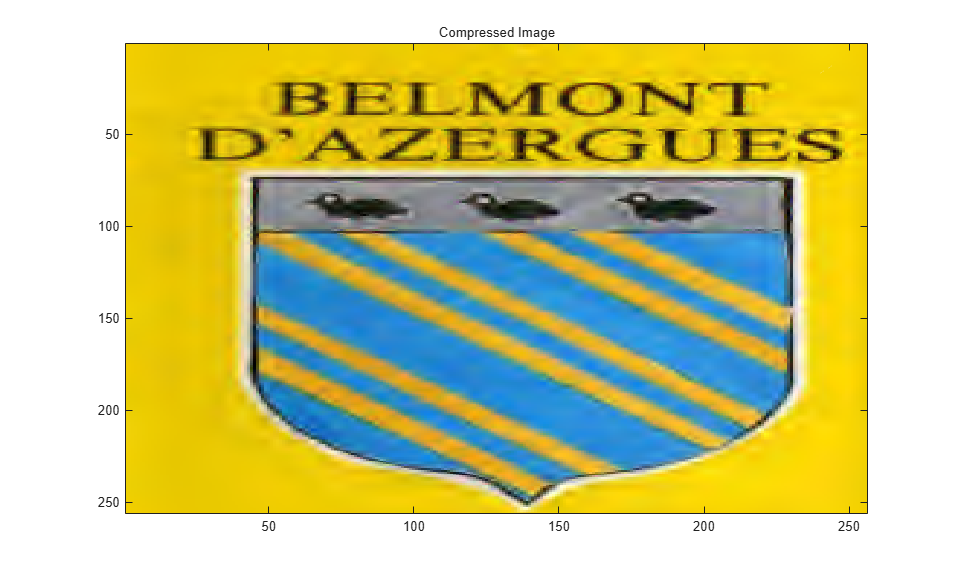This example show how to compress a jpeg image using the adaptively scanned wavelet difference reduction compression method (`'aswdr'`). The conversion color (`'cc'`) uses the Karhunen-Loeve transform (`'kit'`). The maximum number of loops (`'maxloop'`) is set to 11 and the plot type (`'plotpar'`) is set to step through the compression. Show the compression ratio (`cratio`) and the bit-per-pixel ratio (`bpp`), which indicate the quality of the compression.

```[cratio,bpp] = wcompress('c','woodstatue.jpg','woodstatue.wtc', ... 'aswdr','cc','klt','maxloop',11,'plotpar','step'); cratio bpp ```
```cratio = 3.0792 bpp = 0.7390 ```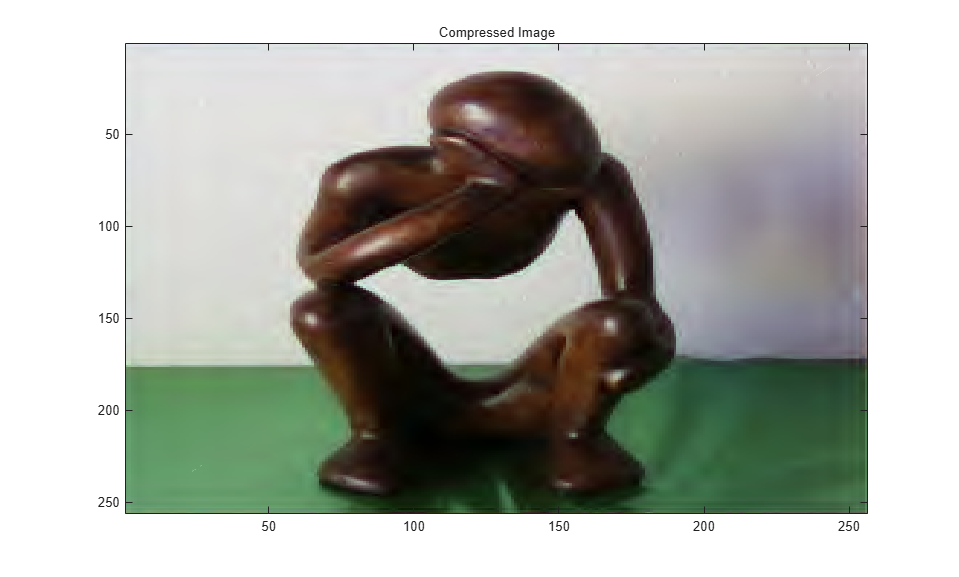Load the compressed image and step through the uncompression process.

```wcompress('u','woodstatue.wtc','step'); ```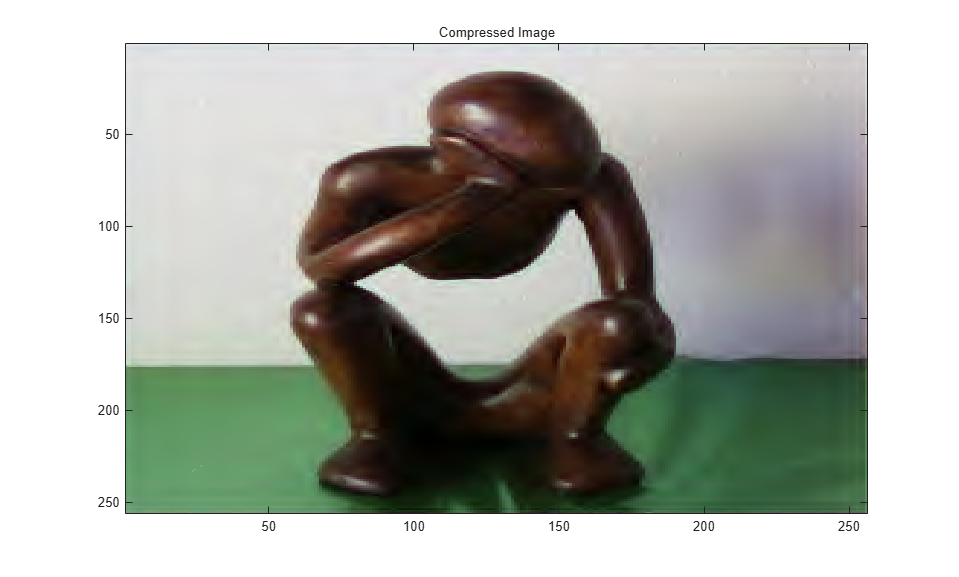This example shows how to compress a grayscale image using the set partitioning in hierarchical trees (`'spiht'`) compression method. It also computes the mean square error (MSE) and the peak signal to noise ratio (PSNR) error values. You use these two measures to quantify the error between two images. The PSNR is expressed in decibels.

Load the image and store it in a file.

```load mask; [cr,bpp] = wcompress('c',X,'mask.wtc','spiht','maxloop',12)```
```cr = 2.8610 ```
```bpp = 0.2289 ```

Load the stored image from the file, uncompress it, and delete the file.

```Xc = wcompress('u','mask.wtc'); delete('mask.wtc')```

Display the original and compressed images.

```colormap(pink(255)) subplot(1,2,1); image(X); title('Original image') axis square subplot(1,2,2); image(Xc); title('Compressed image') axis square```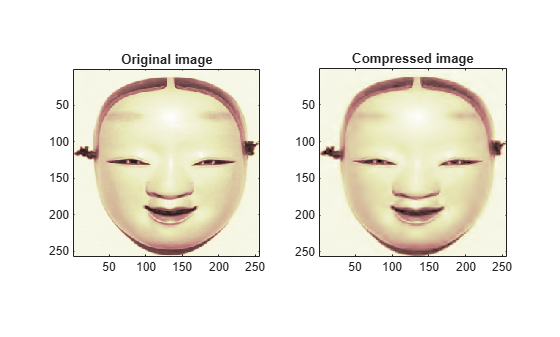Compute the MSE and PSNR.

```D = abs(X-Xc).^2; mse = sum(D(:))/numel(X)```
```mse = 33.6564 ```
`psnr = 10*log10(255*255/mse)`
```psnr = 32.8601 ```

This example shows how to compress a truecolor image using the set partitioning in hierarchical trees - 3D (`'spiht_3D'`) compression method.

Load, compress, and store the image in a file. Plot the original and compressed images. Display the compression ratio (`'cratio'`) and the bits-per-pixel (`'bpp'`), which indicate the quality of the compression.

```load mask; X = imread('wpeppers.jpg'); [cratio,bpp] = wcompress('c',X,'wpeppers.wtc','spiht','maxloop',12)```
```cratio = 1.6549 ```
```bpp = 0.3972 ```
```Xc = wcompress('u','wpeppers.wtc'); delete('wpeppers.wtc')```

Display the original and compressed images.

```subplot(1,2,1) image(X) title('Original image') axis square subplot(1,2,2) image(Xc) title('Compressed image') axis square```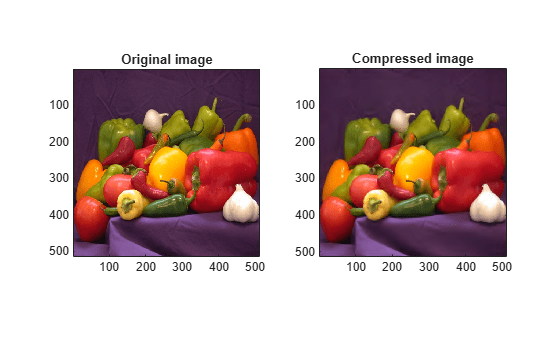Compute the mean square error (MSE) and the peak signal-to-noise ratio (PSNR) error values. You use these two measures to quantify the error between two images. The PSNR is expressed in decibels.

```D = abs(double(X)-double(Xc)).^2; mse = sum(D(:))/numel(X)```
```mse = 26.7808 ```
`psnr = 10*log10(255*255/mse)`
```psnr = 33.8526 ```

## References

Christophe, E., C. Mailhes, P. Duhamel (2006), “Adaptation of zerotrees using signed binary digit representations for 3 dimensional image coding,” EURASIP Journal on Image and Video Processing, 2007, to appear in the special issue on Wavelets in Source Coding, Communications, and Networks, Paper ID 54679.

Misiti, M., Y. Misiti, G. Oppenheim, J.-M. Poggi (2007), Wavelets and their applications, ISTE DSP Series.

Said A., W.A. Pearlman (1996), “A new, fast, and efficient image codec based on set partitioning in hierarchical trees,” IEEE Trans. on Circuits and Systems for Video Technology, Vol. 6, No. 3, pp. 243–250.

Shapiro J.M. (1993), “Embedded image coding using zerotrees of wavelet coefficients”,P IEEE Trans. Signal Proc., Vol. 41, No. 12, pp. 3445–3462.

Strang, G.; T. Nguyen (1996), Wavelets and Filter Banks, Wellesley-Cambridge Press.

Walker J.S. (1999), “Wavelet-Based Image Compression,” University of Wisconsin, Eau Claire, Wisconsin, USA, , Sub-chapter of CRC Press book: Transform and Data Compression. A Primer on Wavelets and Their Scientific Applications.